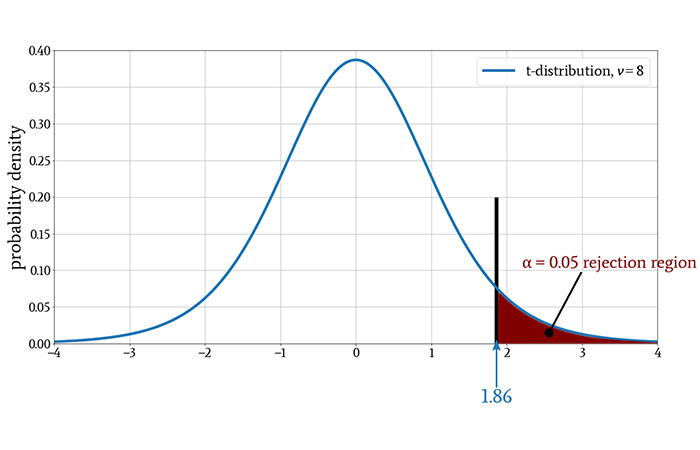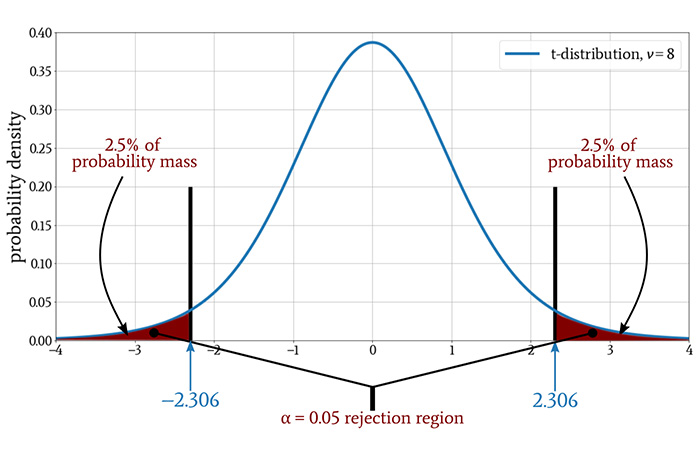Technical Article

# Finding Statistical Significance from t-Tests Applied to Engineered Systems

October 14, 2020 by Robert Keim

## This article discusses important aspects of t-testing with the help of the example experiment presented in the previous article.

Welcome to Robert Keim's series on statistics in electrical engineering. As we near the end of the series, you may be wondering about the conceptual building blocks that lead to this point. If you'd like to catch up, please browse the list of preceding articles below. Otherwise, skip to the next section to learn more about how we can derive statistical significance from t-tests applied to engineered systems.

### A Review of Our Calculated t-Values

As reported in the previous article, we obtained the following measurements during our imaginary experiment involving operating temperature and packet error rate (PER):

PER
0.0010290
0.0010113
0.0010380
0.0010198
0.0009702
0.0010486
0.0010503
0.0009941
0.0010067

After calculating the sample mean and the sample standard deviation, we determined that t = 2.13. Since the critical value was t* = 1.86, we found that t > t*, and consequently we rejected the null hypothesis.

### One-Tailed vs. Two-Tailed Testing

One questionable aspect of our experiment was the assumption that increasing the temperature would lead only to equal or worse PER performance. Because of this assumption, our analysis did not consider the possibility of higher temperature being correlated with improved PER, and this is reflected in the use of a one-tailed test:Is this assumption valid? Temperatures that are significantly higher than room temperature tend to cause electronic circuits to behave in a way that is, overall, less desirable.

However, the relationship between temperature and system performance is influenced by various factors that interact in potentially complex ways. Furthermore, our example is built around a wireless communication system, and the behavior of RF circuitry is particularly difficult to predict.

Thus, we might decide to design the experiment differently. Since we’re going to all the trouble of heating up the lab, setting up the system, collecting data, and so forth, maybe it makes sense to look for evidence that increased temperature can cause a statistically significant change in PER.

We’re not just looking for degraded PER anymore. Now we’re assuming that the increased operating temperature can result in a higher PER or a lower PER, and this means that we need a two-tailed test.

A two-tailed test with the same significance level has the same amount of probability mass in the rejection region, but the region is split into two sections, one above the mean and the other below the mean. Consequently, the critical value will change:Something interesting has happened: our t-value of 2.13 is not greater than the critical value! In other words, our analysis now indicates that the experiment did not demonstrate a relationship between temperature and PER.

This exercise gives us two things to think about. First, we need to be careful about assumptions that lead us to a one-tailed or two-tailed test, because these assumptions can be determining factors in our assessment of statistical significance.

Second, significance testing is not a rock-solid, purely mathematical procedure. In addition to the choice of one-tailed or two-tailed testing, we have the significance threshold itself, which is rather arbitrary. It’s true that the two-tailed test moved the t-value out of the rejection region, but we could move it back into the rejection region by choosing ⍺ = 0.1 instead of ⍺ = 0.05.

### The Effect of Sample Size on Statistical Significance

Rejection of the null hypothesis occurs when the t-value is greater than the critical value. Thus, if our objective is to demonstrate statistical significance, we want a higher t-value. Let’s take another look at the equation that we use to calculate t-values:

$t=\frac{\bar{x}-\mu}{s/\sqrt{n}}$

If we increase the sample size (denoted by n), the quantity s/√n decreases, and this causes the t-value to increase. Thus, if we want a higher t-value, all we need to do is increase the sample size.

For example: If I take the same exact PER measurements but replicate the data set five times (such that n = 54), the t-value increases from the original t = 2.13 to t = 5.48. If we collect more data, we increase the t-value even when the new measurements don’t create a noteworthy change in mean or standard deviation.

To make matters worse, the critical value decreases as sample size increases. With n = 9, we had ν = 8 and t* = 1.860. With n = 54, we have ν = 53 and t* = 1.674. In general, larger sample sizes make statistical significance easier to achieve, because they tend to result in higher t-values and lower critical values.

This is a known problem with statistical analysis in which we compute a p-value and compare it to a significance level. You can read more about this issue in a journal article entitled “Using Effect Size—or Why the P Value Is Not Enough.” The article points out that a very large sample size is likely to lead to a statistically significant p-value even when the real-life effect is negligible.

### Conclusion

I hope that this article and the previous article have helped you to understand how a t-test can be useful when you’re characterizing or troubleshooting an electronic system. It’s also good to remember that statistical significance has its limitations.

Maybe in a future article, we’ll discuss effect size, which is not influenced by sample size and functions as an important complement to statistical significance.